# Customers Grouped by Count of Their Orders – Static Segmentation in Power BIOne of the interesting calculation challenges in BI systems is a segmentation or grouping on a numeric value, based on an occurrence of another value. As an example; You might want to know How many customers ordered once, twice, three times or more. The challenge is that the data in the transactional table is not aggregated, and this type of calculation needs two aggregations. There are two ways of calculating such a thing in Power BI; statically or dynamically. In this article, I’ll explain the static method for this calculation.

## Segmentation: Group By a Count of Values

Let’s go through this challenge using an example. Below, I have the FactInternetSales table, which has the information on all sales transactions made. the Sales Order Number and the order line number are two columns in the table, as well as the CustomerKey.The problem we want to solve using the data above is that:

How many customers did only one order, how many two times, how many three times and so on?

something like the below chart as the output:This doesn’t seem to be a complex solution. You need to have a field which is the number of orders for each customer, and then get the count of customers for each group. The fact that the data in the table is not aggregated, however, makes it a bit of a challenge.

If the data in the table, was like this, it was much easier to get the result out:Because then you would simply use the Count of Orders as an axis, and then Count of CustomerKey as the value of the chart.

However, since that table does not exist, we need to create it.

## Static Grouping

In Power BI, there are multiple ways of achieving the result. The method used in this article is what I call Static Segmentation or Grouping. Because the calculation in this method is pre-calculated. In the next article, I’ll explain how to do it dynamically.

## Grouping in DAX

You can use any of the functions that are good for grouping in DAX, such as GroupBy, Summarize, SummarizeColumns, etc. Let’s say I want to use the Summarize option. Before using that option, I create a measure that I use to get the count of orders as below:

`Count of Orders = COUNTROWS(VALUES(FactInternetSales[SalesOrderNumber]))`

Because In the FactInternetSales table I expect sometimes multiple records per SalesOrderNumber, the expression above gives me the unique number of orders. (Values gives me the unique list of SalesOrderNumber, and the CountRows gives me the count of that unique list)Now, using creating a new table, You can calculate the count of orders for each customer,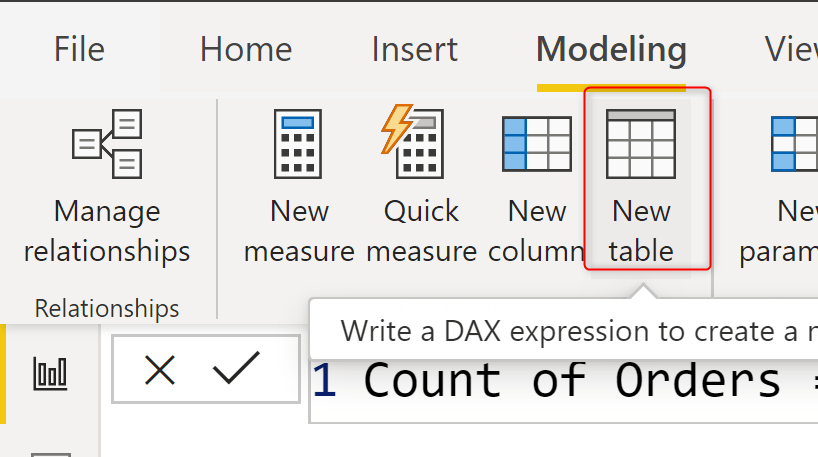The expression below is using Summarize to group the data of FactInternetSales by CustomerKey field, and return the Count of Orders for each CustomerKey;

```Customer Orders =
SUMMARIZE(
FactInternetSales,
FactInternetSales[CustomerKey],
'Count of Orders',
[Count of Orders]
)```

The output table looks like below:This calculation can be done also using other DAX functions such as GroupBy, SummarizeColumns, etc.

Now that this column is available, you can use the Count of Orders as the Axis of a chart, and CustomerKey as the value, which then will result in calculating the Count of CustomerKey as the value.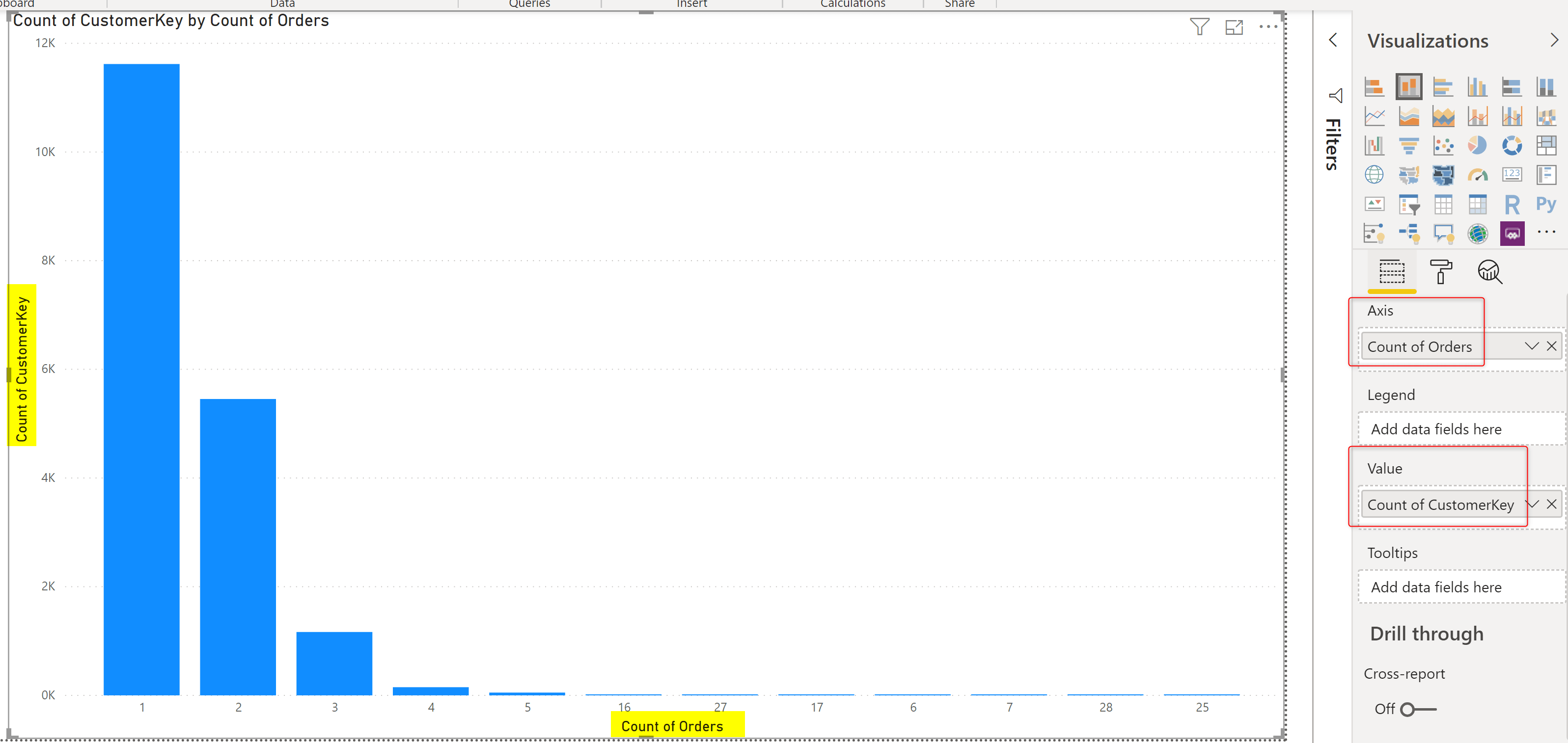The chart above also has the Type of X-Axis as Categorical in the format section to look like what you see in the above screenshot.

## Relationship to the Detailed Table

This newly created table is an aggregated table and is good to be related to the main detailed table because then you can select a group (let’s say those who only had three sales orders), and see how much in total they purchased, and see their customer number and other details.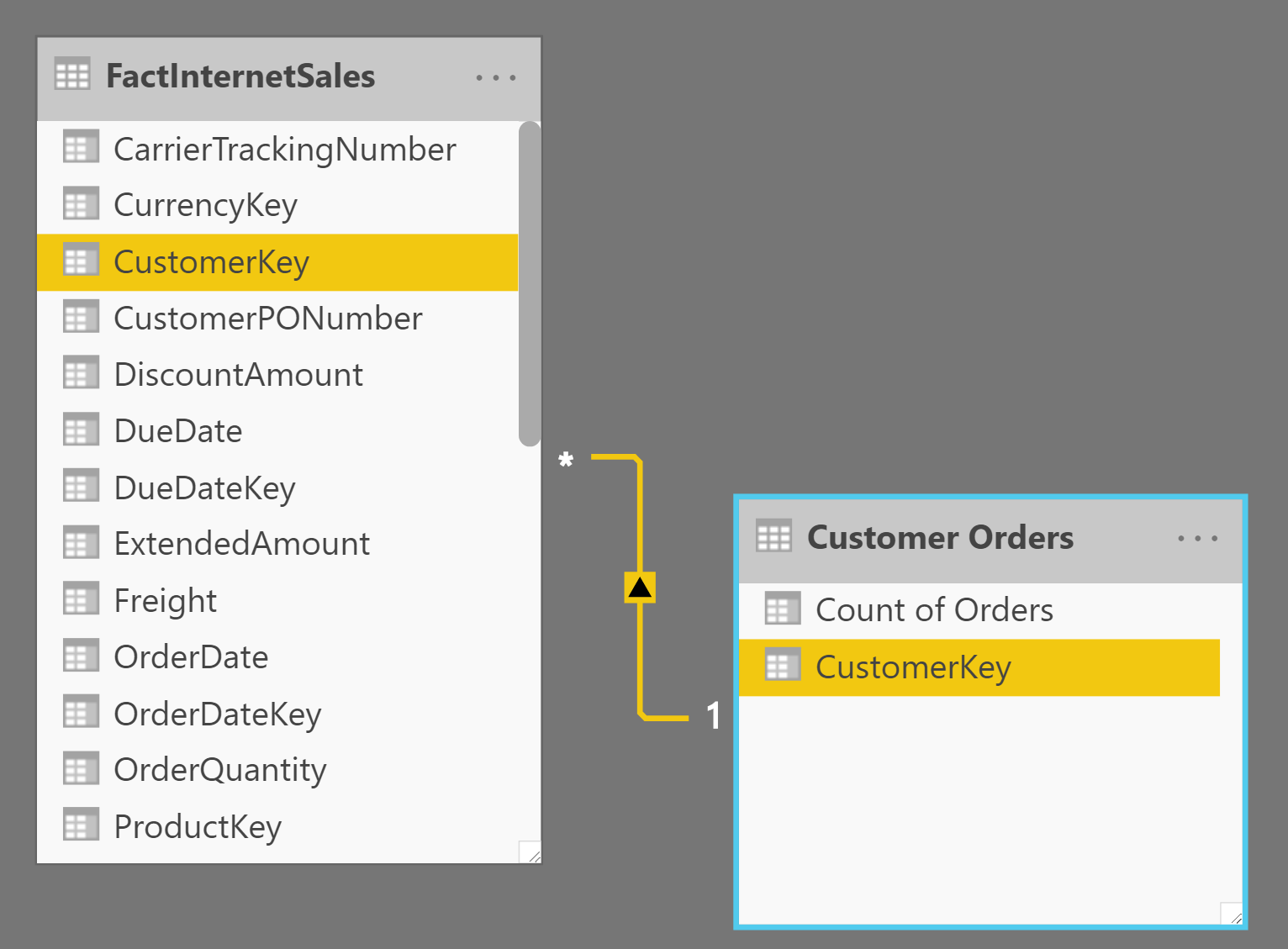Now, I can even create pages of data visualization like below: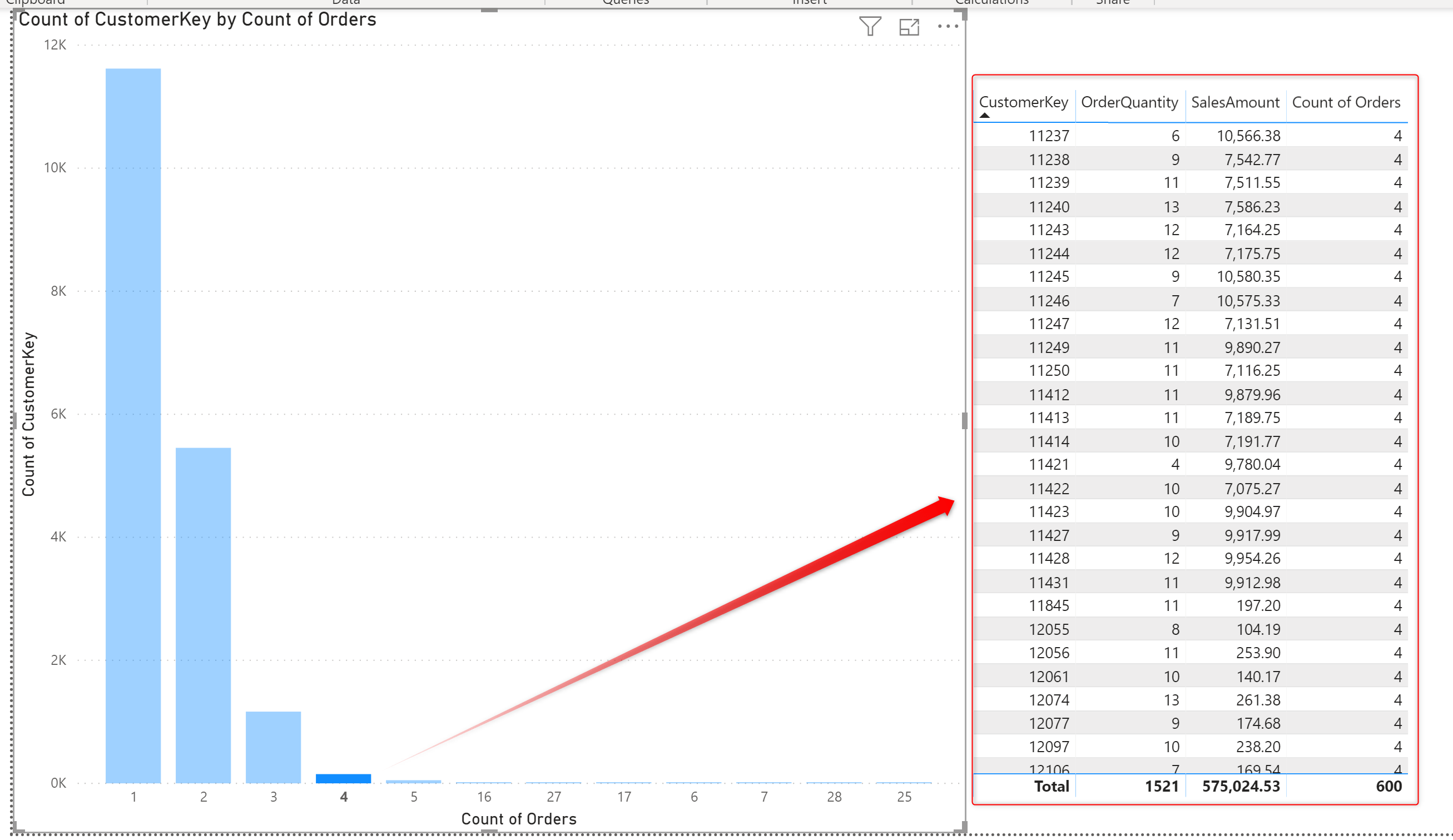## Grouping in Power Query

The same result can be achieved using the Grouping in Power Query, and it would even be possible with writing a few lines of code! First, you can create a reference from the main table;Note that the above should be done in the Transform Data, or Edit Queries window.

Then use the Group By on the newly created reference table;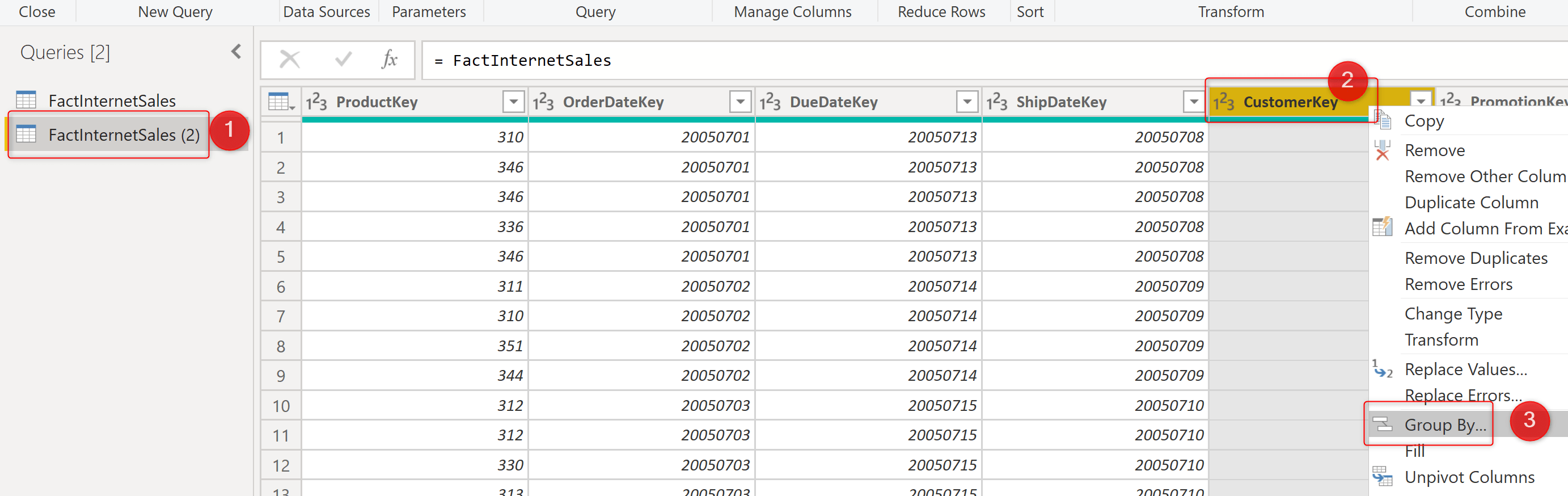Since the Discount Count by a column is not a default aggregation option, you can just select Discount Count Rows;And then edit the formula, and change the bit below:

`= Table.Group(Source, {'CustomerKey'}, {{'Count of Orders', each Table.RowCount(Table.Distinct(_)), type number}})`

with below;

`= Table.Group(Source, {'CustomerKey'}, {{'Count of Orders', each Table.RowCount(List.Distinct(_[SalesOrderNumber])), type number}})`

The technique above will give you the distinct count as below;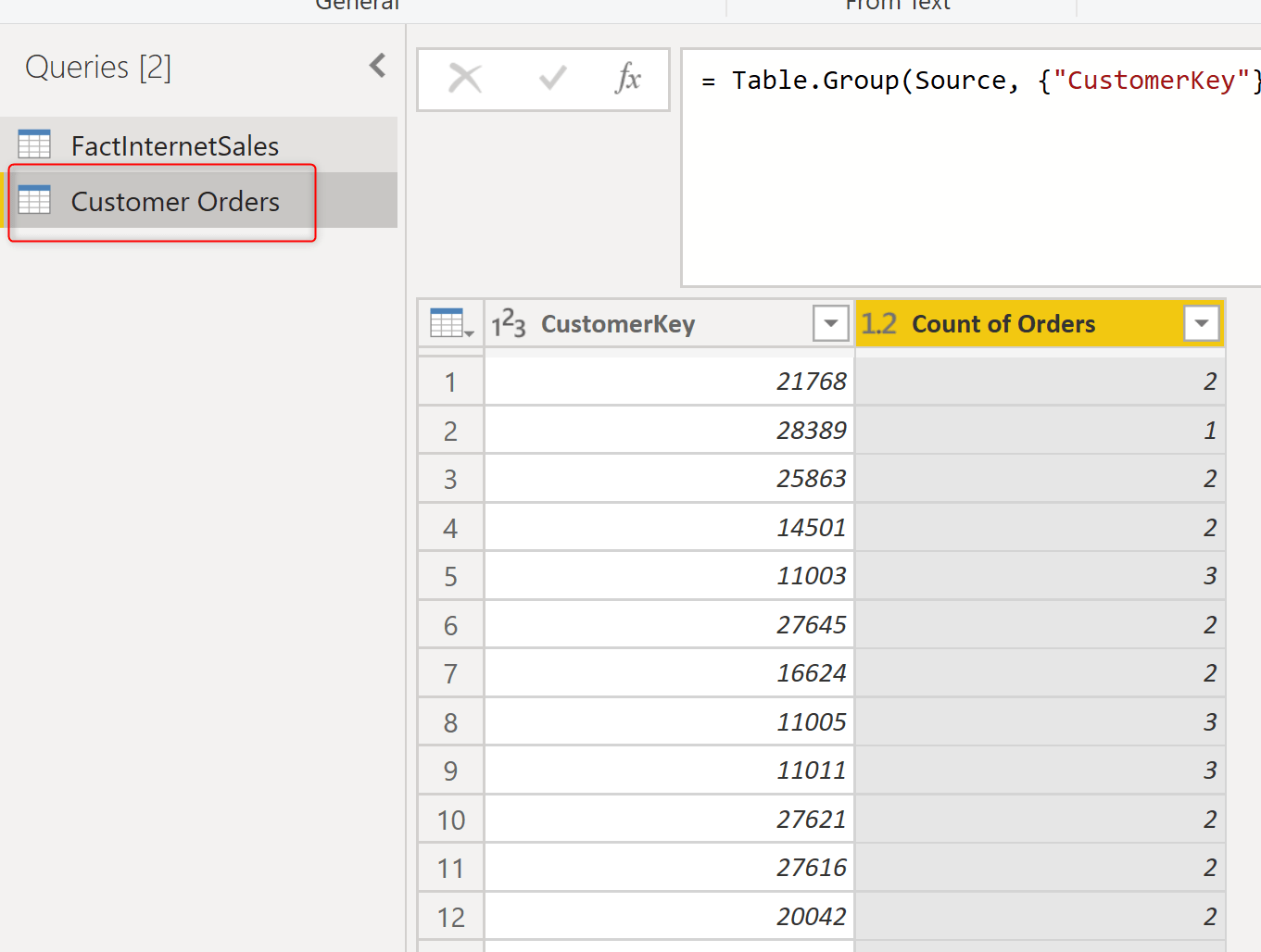Similar to the other example, this table can be related to the FactInternetSales table, and then be used in the visualization.

## Which method is better?

Both the DAX method and the Power Query method are good in this example. However, I personally prefer to keep all pre-calculations in the Power Query, unless using a DAX function gives me some useful ways of achieving something really fast (such as Path functions in DAX).

## Pros and Cons of Static Grouping

Grouping as mentioned above is defined statically, which means that the calculation happened at the time of refreshing the data (either using the DAX calculated table or using Power Query grouping). This method won’t have any performance problems, because the calculation happens at the time of refreshing the data, and the user won’t be impacted by how long the calculation takes to run. The calculation of transformations in Power Query or DAX calculated table are all happening at the time of refreshing the report;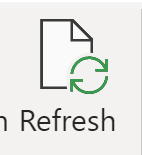Because the calculation is done before the refresh time, the calculation is not aware of the user’s selection of slicers. For example, if you want to do the calculation only within the range that user has selected in a date slicer in the report, this method mentioned in this article, won’t be the answer for that;If you are doing a pre-calculation, you need to consider all combinations. Or, alternatively, you can use a dynamic segmenting or grouping using a measure which is what I will explain in the next blog article.

## Dynamic Segmentation

If you want to see how the segmentation can be implemented in a dynamic way, check out this article.

Trainer, Consultant, Mentor
Reza Rad is a Microsoft Regional Director, an Author, Trainer, Speaker and Consultant. He has a BSc in Computer engineering; he has more than 20 years’ experience in data analysis, BI, databases, programming, and development mostly on Microsoft technologies. He is a Microsoft Data Platform MVP for 12 continuous years (from 2011 till now) for his dedication in Microsoft BI. Reza is an active blogger and co-founder of RADACAD. Reza is also co-founder and co-organizer of Difinity conference in New Zealand, Power BI Summit, and Data Insight Summit.
Reza is author of more than 14 books on Microsoft Business Intelligence, most of these books are published under Power BI category. Among these are books such as Power BI DAX Simplified, Pro Power BI Architecture, Power BI from Rookie to Rock Star, Power Query books series, Row-Level Security in Power BI and etc.
He is an International Speaker in Microsoft Ignite, Microsoft Business Applications Summit, Data Insight Summit, PASS Summit, SQL Saturday and SQL user groups. And He is a Microsoft Certified Trainer.
Reza’s passion is to help you find the best data solution, he is Data enthusiast.
His articles on different aspects of technologies, especially on MS BI, can be found on his blog: https://radacad.com/blog.

## 2 thoughts on “Customers Grouped by Count of Their Orders – Static Segmentation in Power BI”

•Alberto says:

Hello,
in the Dax solution, it’s very likely to have a Customer dimension table in the model.
In such scenario, what about creating a calculated column directly in the Customer tables ?

•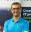Reza Rad says:

Hi Alberto
Creating the calculated column in the customer table is also a solution to consider for sure. In this scenario, I wanted to explain if there is no other tables, how the segmentation works in general.
Cheers
Reza